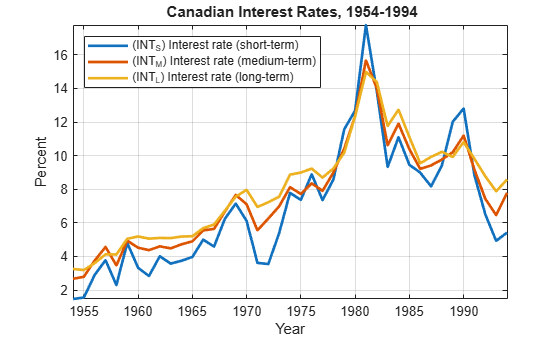# Test for Cointegration Using the Engle-Granger Test

This example shows how to test the null hypothesis that there are no cointegrating relationships among the response series composing a multivariate model.

Load Data_Canada into the MATLAB® Workspace. The data set contains the term structure of Canadian interest rates . Extract the short-term, medium-term, and long-term interest rate series.

load Data_Canada Y = Data(:,3:end); % Multivariate response series

Plot the response series.

figure plot(dates,Y,'LineWidth',2) xlabel 'Year'; ylabel 'Percent'; names = series(3:end); legend(names,'location','NW') title '{\bf Canadian Interest Rates, 1954-1994}'; axis tight grid onThe plot shows evidence of cointegration among the three series, which move together with a mean-reverting spread.

To test for cointegration, compute both the $\tau$ (t1) and $z$ (t2) Dickey-Fuller statistics. egcitest compares the test statistics to tabulated values of the Engle-Granger critical values.

[h,pValue,stat,cValue] = egcitest(Y,'test',{'t1','t2'})
h = 1x2 logical array 0 1 
pValue = 1×2 0.0526 0.0202 
stat = 1×2 -3.9321 -25.4538 
cValue = 1×2 -3.9563 -22.1153 

The $\tau$ test fails to reject the null of no cointegration, but just barely, with a p-value only slightly above the default 5% significance level, and a statistic only slightly above the left-tail critical value. The $z$ test does reject the null of no cointegration.

The test regresses Y(:,1) on Y(:,2:end) and (by default) an intercept c0. The residual series is

[Y(:,1) Y(:,2:end)]*beta - c0 = Y(:,1) - Y(:,2:end)*b - c0.

The fifth output argument of egcitest contains, among other regression statistics, the regression coefficients c0 and b.

Examine the regression coefficients to examine the hypothesized cointegrating vector beta = [1; -b].

[~,~,~,~,reg] = egcitest(Y,'test','t2'); c0 = reg.coeff(1); b = reg.coeff(2:3); beta = [1;-b]; h = gca; COrd = h.ColorOrder; h.NextPlot = 'ReplaceChildren'; h.ColorOrder = circshift(COrd,3);plot(dates,Y*beta-c0,'LineWidth',2); title '{\bf Cointegrating Relation}'; axis tight; legend off; grid on;The combination appears relatively stationary, as the test confirms.